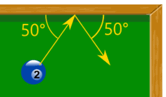# Momentum

Momentum is how much something wants to keep moving in the same direction.

This truck would be hard to stop ...... it has a lot of momentum.

Faster? More momentum!
Heavier?
More momentum!

Momentum is mass times velocity.
The symbol is p:

p = m v### Example: What is the momentum of a 1500 kg car going at highway speed of 28 m/s (about 100 km/h or 60 mph)?

p = m v

p = 1500 kg × 28 m/s

p = 42,000 kg m/s

The unit for momentum is:

• kg m/s (kilogram meter per second), or
• N s (Newton second)

They are the same! 1 kg m/s = 1 N s

We will use both here.

More examples:

Mass Speed Momentum 7.5 g 0.0075 kg 1000 m/s 0.0075 × 1000 = 7.5 kg m/s 57 g 0.057 kg 50 m/s 0.057 × 50 = 2.85 kg m/s 16 oz 0.45 kg 100 km/h 28 m/s 0.45 × 28 = 12.6 kg m/s 22 oz 0.6 kg 3 m/s 0.6 × 3 = 1.8 kg m/s 400 g 0.4 kg 7 m/s 0.4 × 7 = 2.8 kg m/s 80 kg 9 km/h 2.5 m/s 80 × 2.5 = 200 kg m/s 1500 kg 100 km/h 28 m/s 1500 × 28 = 42,000 kg m/s

Momentum has direction: the exact same direction as the velocity.

But many examples here only use speed (velocity without direction) to keep it simple.### Animation

Play with momentum in this animation.

## Impulse

Impulse is change in momentum. Δ is the symbol for "change in", so:

Impulse is Δp

Force can be calculated from the change in momentum over time (called the "time rate of change" of momentum):

F = Δp Δt### Example: You are 60 kg and run at 3 m/s into a wall. The wall stops you in 0.05 s. What is the force? The wall is then padded and stops you in 0.2 s. What is the force?

First calculate the impulse:

Δp = m v

Δp = 60 kg x 3 m/s

Δp = 180 kg m/s

Stopping in 0.05 s:

F = Δp Δt

F = 180 kg m/s 0.05 s = 3600 N

Stopping in 0.2 s:

F = Δp Δt

F = 180 kg m/s 0.2 s = 900 N

Stopping at a slower rate has much less force!

• And that is why padding works so well
• And also why crash helmets save lives
• And why cars have crumple zones

### Q: Isn't force normally calculated using F = ma ? A: Well, F = Δp Δt is the same thing, just a different form:

 Start with: F = ma Acceleration is change in velocity v over time t: F = m Δv Δt Rearrange to: F = Δmv Δt And Δmv is change in momentum: F = Δp Δt

## Impulse From Force

We can rearrange:

F = Δp Δt

Into:

Δp = F Δt

So we can calculate the Impulse (the change in momentum) from force applied for a period of time.

### Example: A ball is hit with a 300 N force. High speed cameras show the contact lasted for 0.02 s. What was the impulse?

Δp = F Δt

Δp = 300 N × 0.02 s

Δp = 6 N s

## Momentum is Conserved

Conserved: the total stays the same (within a closed system).Closed System: where nothing transfers in or out, and no external force acts on it.

### In our Universe:

• Mass is conserved (it can change form, be moved around, cut up or joined together, but the total mass stays the same over time)
• Energy is conserved (it also can change form, to light, to heat and so on)
• And Momentum is also conserved!

Note: At an atomic level Mass and Energy can be converted via E=mc2, but nothing gets lost.

## Momentum is a Vector

Momentum is a vector: it has size AND direction.Sometimes we don't mention the direction, but other times it is important!

### One Dimension

A question may have only one dimension, and all we need is positive or negative momentum:### Two or More Dimensions

Questions can be in two (or more) dimensions like this one:### Example: A pool ball bounces! It hits the edge with a velocity of 8 m/s at 50°, and bounces off at the same speed and reflected angle. It weighs 0.16 kg. What is the change in momentum?

Let's break the velocity into x and y parts. Before the bounce:

• vx = 8 × cos(50°)   ...going along
• vy = 8 × sin(50°)   ...going up

After the bounce:

• vx = 8 × cos(50°)   ...going along
• vy = 8 × −sin(50°)   ...going down

The x-velocity does not change, but the y-velocity changes by:

Δvy = (8+8) × sin(50°)
= 16 × sin(50°)

And the change in momentum is:

Δp = m Δv

Δp = 0.16 kg × 16 × sin(50°) m/s

Δp = 1.961... kg m/s

### Footnote: The formula

p = m v
Momentum is mass times velocity

is not the full story!

It is a wonderful and useful formula for normal every day use, but when we look at the atomic scale things don't actually collide. They interact from a distance through electro-magnetic fields.

And the interaction does not need mass, because light (which has no mass) can have momentum.

11985, 17629, 11991, 17630, 17636, 11993, 17635, 17640, 17643, 17648